# ggrepel

## Motivation

Some text labels overlap each other in plots created with geom_text:

library(ggplot2)
ggplot(mtcars) +
geom_point(aes(wt, mpg), color = 'red') +
geom_text(aes(wt, mpg, label = rownames(mtcars))) +
theme_classic(base_size = 16)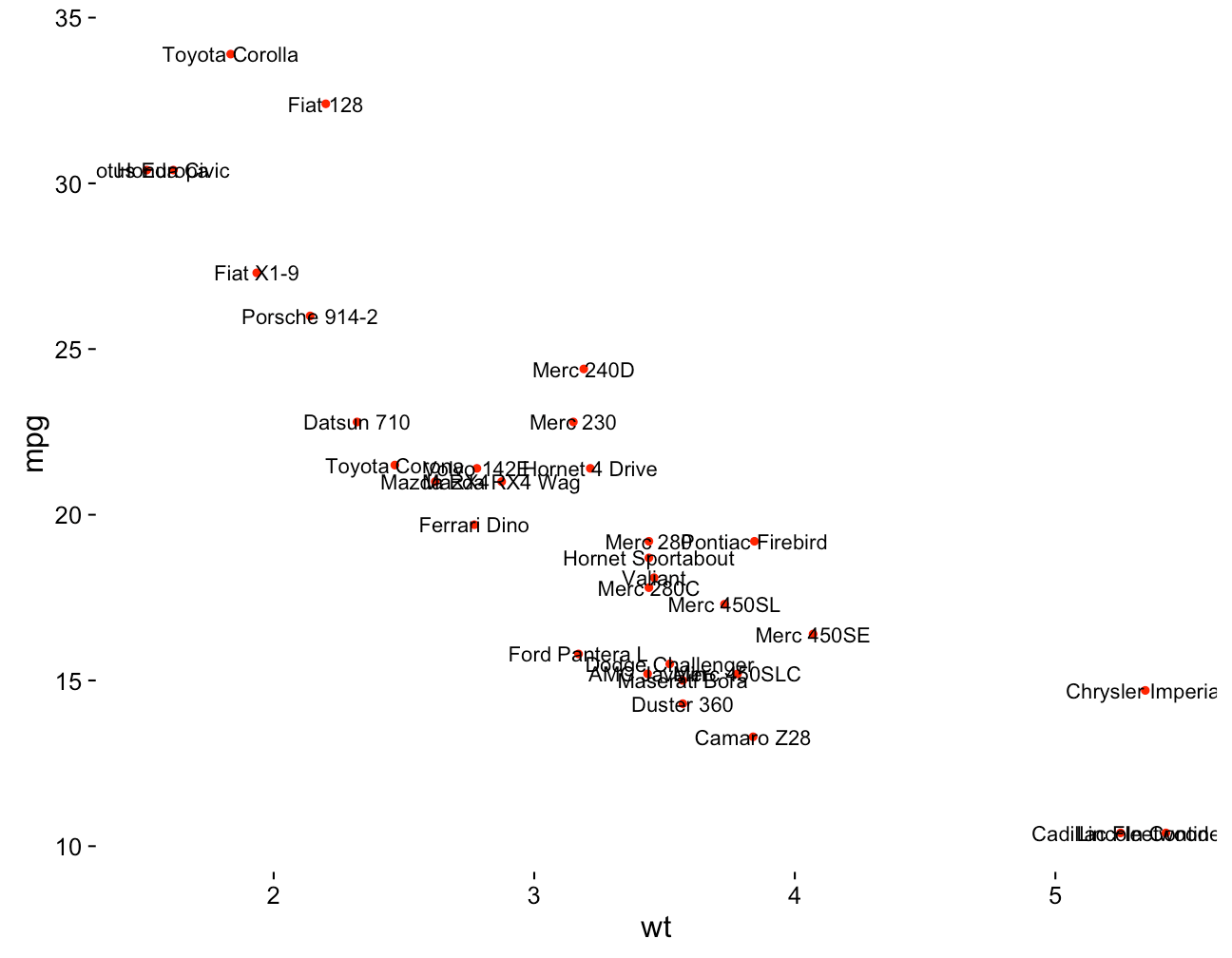## Algorithm

ggrepel implements functions to repel overlapping text labels away from each other and away from the data points that they label. The algorithm works as follows:

• For each box:
• Move the box into the allowed plotting area.
• If the bounding box overlaps other boxes:
• Repel the overlapping boxes from each other.
• If the bounding box overlaps data points:
• Repel the box away from the data points.
• Repeat until all overlaps are resolved, up to a preset limit of iterations.

## Usage Examples

### geom_text_repel

We can repel the text labels away from each other by loading ggrepel and using geom_text_repel instead:

library(ggrepel)
set.seed(42)
ggplot(mtcars) +
geom_point(aes(wt, mpg), color = 'red') +
geom_text_repel(aes(wt, mpg, label = rownames(mtcars))) +
theme_classic(base_size = 16)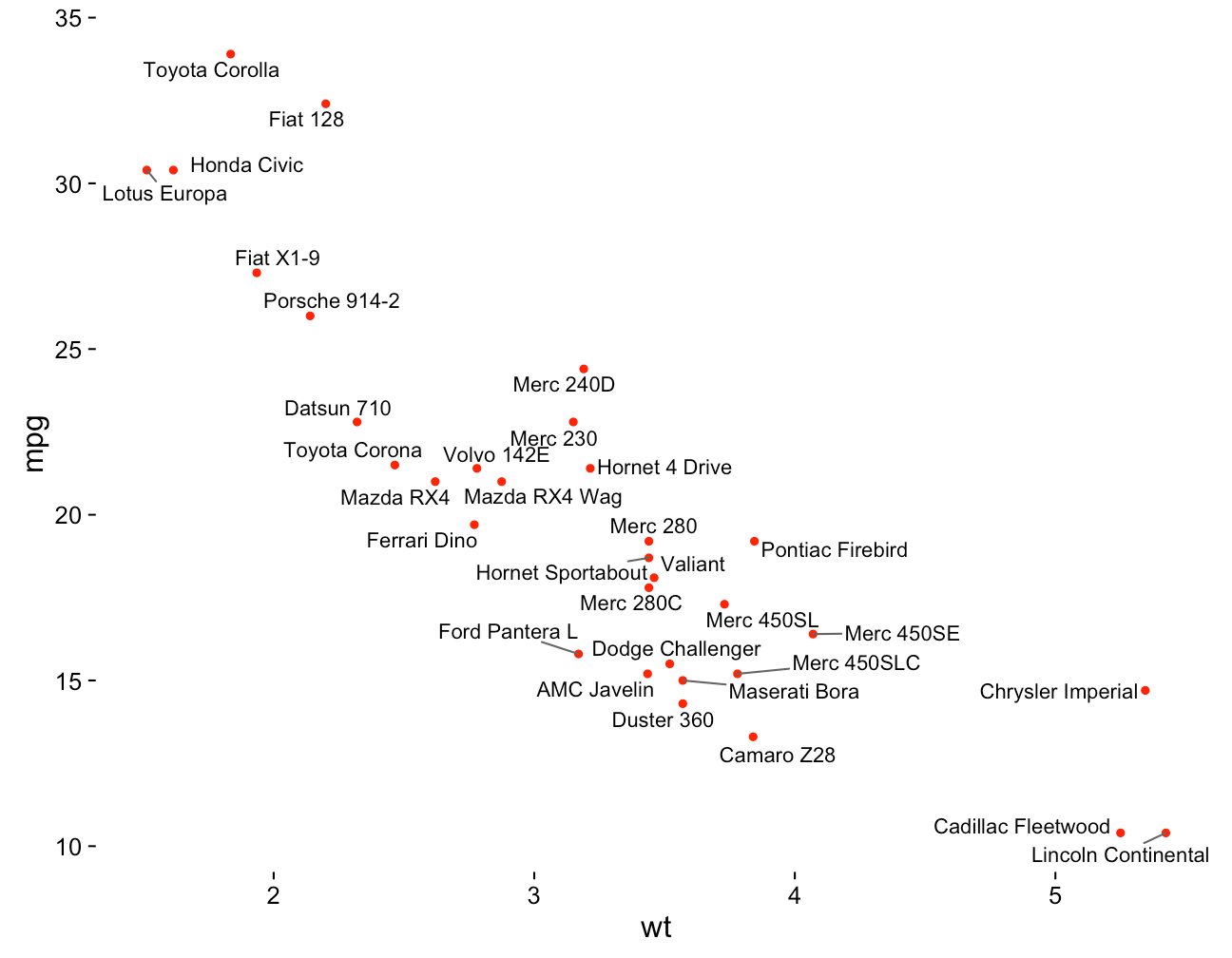### geom_label_repel

geom_label_repel is based on geom_label.

set.seed(42)
ggplot(mtcars) +
geom_point(aes(wt, mpg), size = 5, color = 'grey') +
geom_label_repel(
aes(wt, mpg, fill = factor(cyl), label = rownames(mtcars)),
fontface = 'bold', color = 'white',
) +
theme_classic(base_size = 16)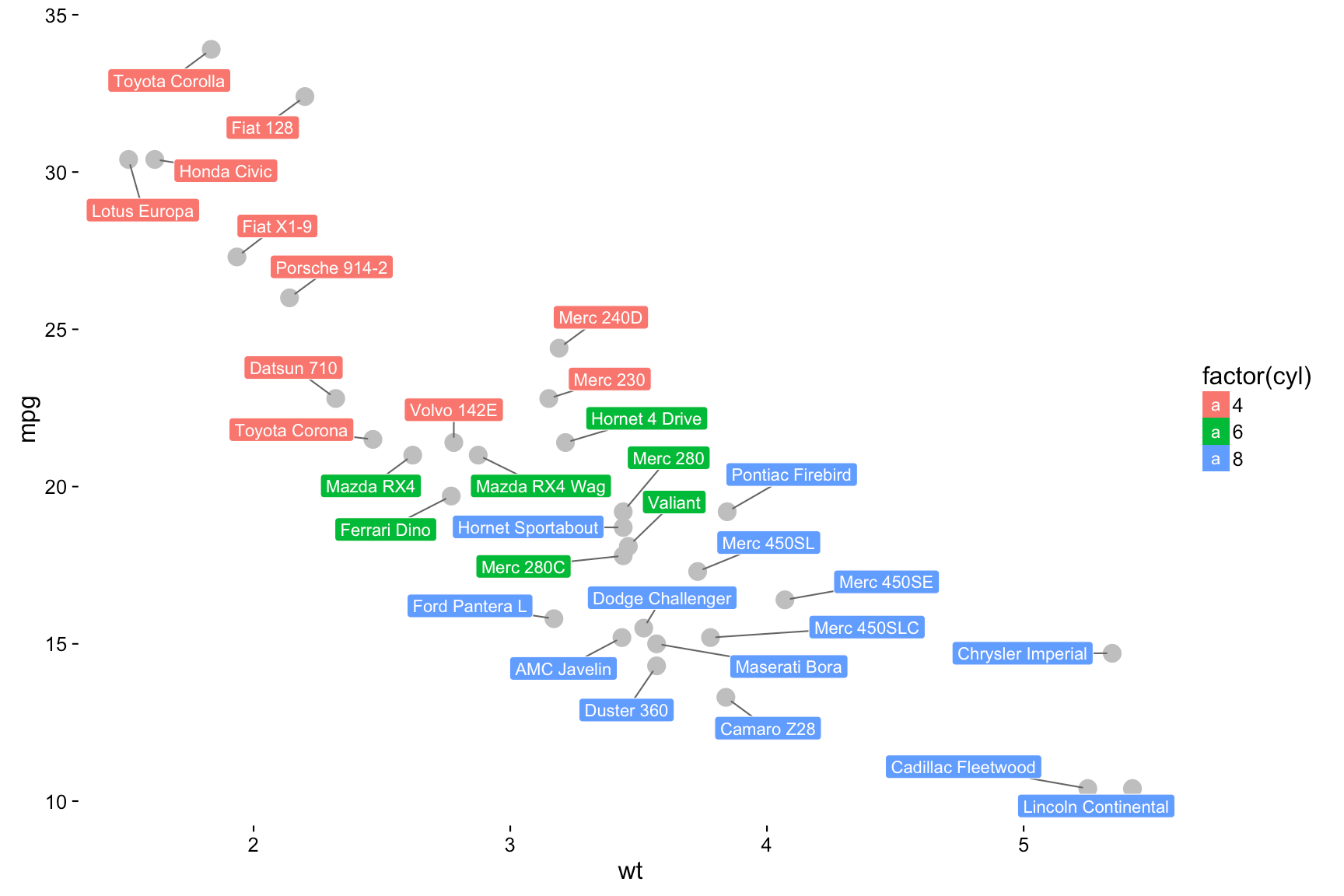### Options

All options available for geom_text such as size, angle, family, fontface are also available for geom_text_repel.

However, the following parameters are not supported:

• hjust
• vjust
• position
• check_overlap

ggrepel provides additional parameters for geom_text_repel and geom_label_repel:

• segment.color is the line segment color
• segment.size is the line segment thickness
• segment.alpha is the line segment transparency
• box.padding is the padding surrounding the text bounding box
• point.padding is the padding around the labeled point
• arrow is the specification for arrow heads created by grid::arrow
• force is the force of repulsion between overlapping text labels
• max.iter is the maximum number of iterations to attempt to resolve overlaps
• nudge_x is how much to shift the starting position of the text label along the x axis
• nudge_y is how much to shift the starting position of the text label along the y axis

Here is an example that uses all of these options:

set.seed(42)
ggplot(mtcars) +
geom_point(aes(wt, mpg, color = factor(cyl)), size = 3) +
geom_text_repel(
aes(
wt, mpg,
color = factor(cyl),
label = rownames(mtcars),
# Cars with 4 cylinders are rotated 90 degrees.
angle = ifelse(mtcars$cyl == 4, 90, 0) ), size = 4, family = 'Times', fontface = 'bold', # Add extra padding around each text label. box.padding = unit(0.5, 'lines'), # Add extra padding around each data point. point.padding = unit(1.6, 'lines'), # Color of the line segments. segment.color = '#cccccc', # Width of the line segments. segment.size = 0.5, # Draw an arrow from the label to the data point. arrow = arrow(length = unit(0.01, 'npc')), # Strength of the repulsion force. force = 1, # Maximum iterations of the naive repulsion algorithm O(n^2). max.iter = 3e3, # Cars with 6 cylinders are nudged up and to the right. nudge_x = ifelse(mtcars$cyl == 6, 2, 0),
nudge_y = ifelse(mtcars$cyl == 6, 9, 0) ) + scale_color_discrete(name = 'cyl') + scale_x_continuous(expand = c(0.5, 0)) + scale_y_continuous(expand = c(0.25, 0)) + theme_classic(base_size = 16)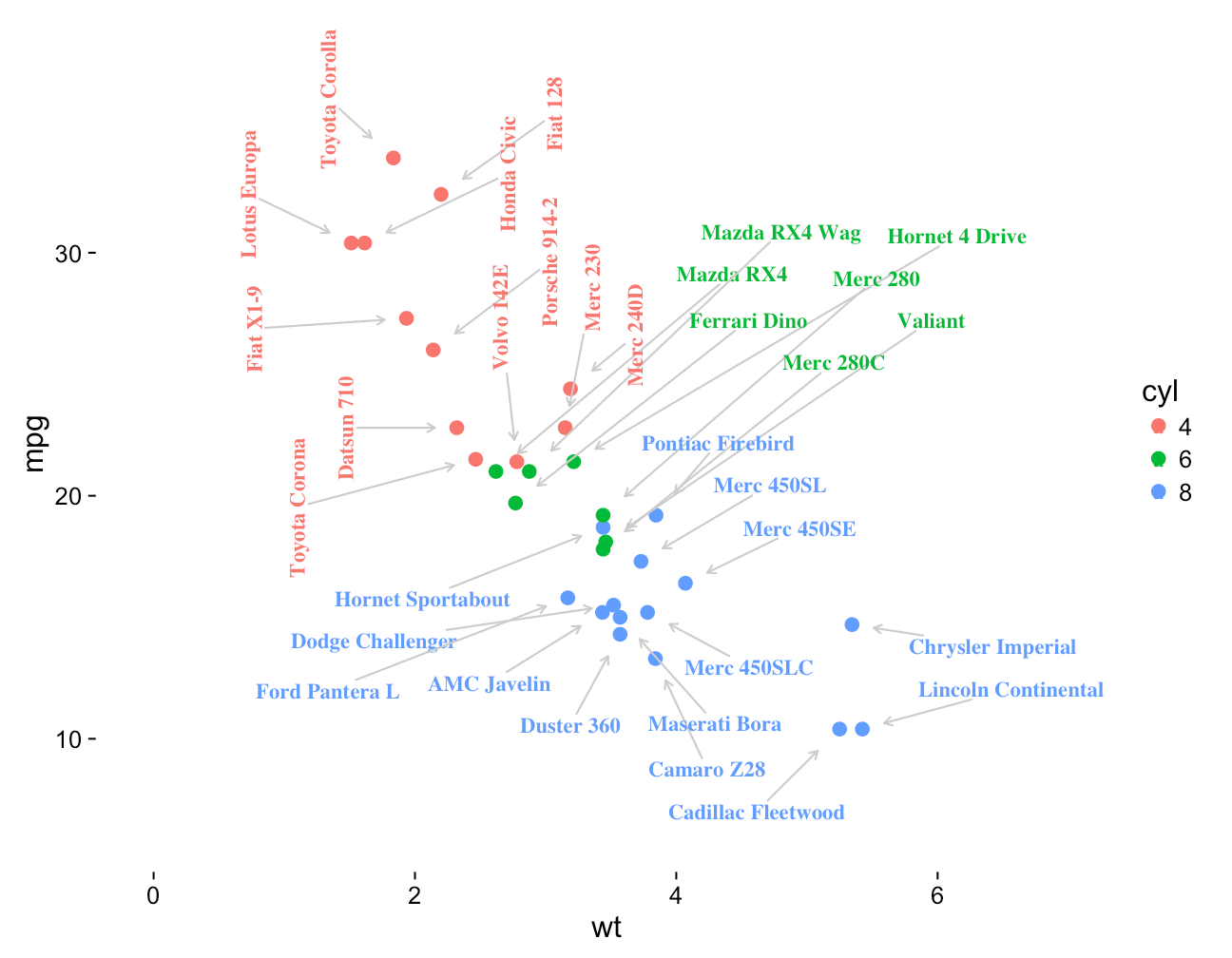### Repel labels and ignore data points Set point.padding = NA to exclude all data points from repulsion calculations. set.seed(42) mtcars$label <- rownames(mtcars)

ggplot(mtcars, aes(wt, mpg, label = label)) +
geom_point(color = "red") +
theme_bw(base_size = 16)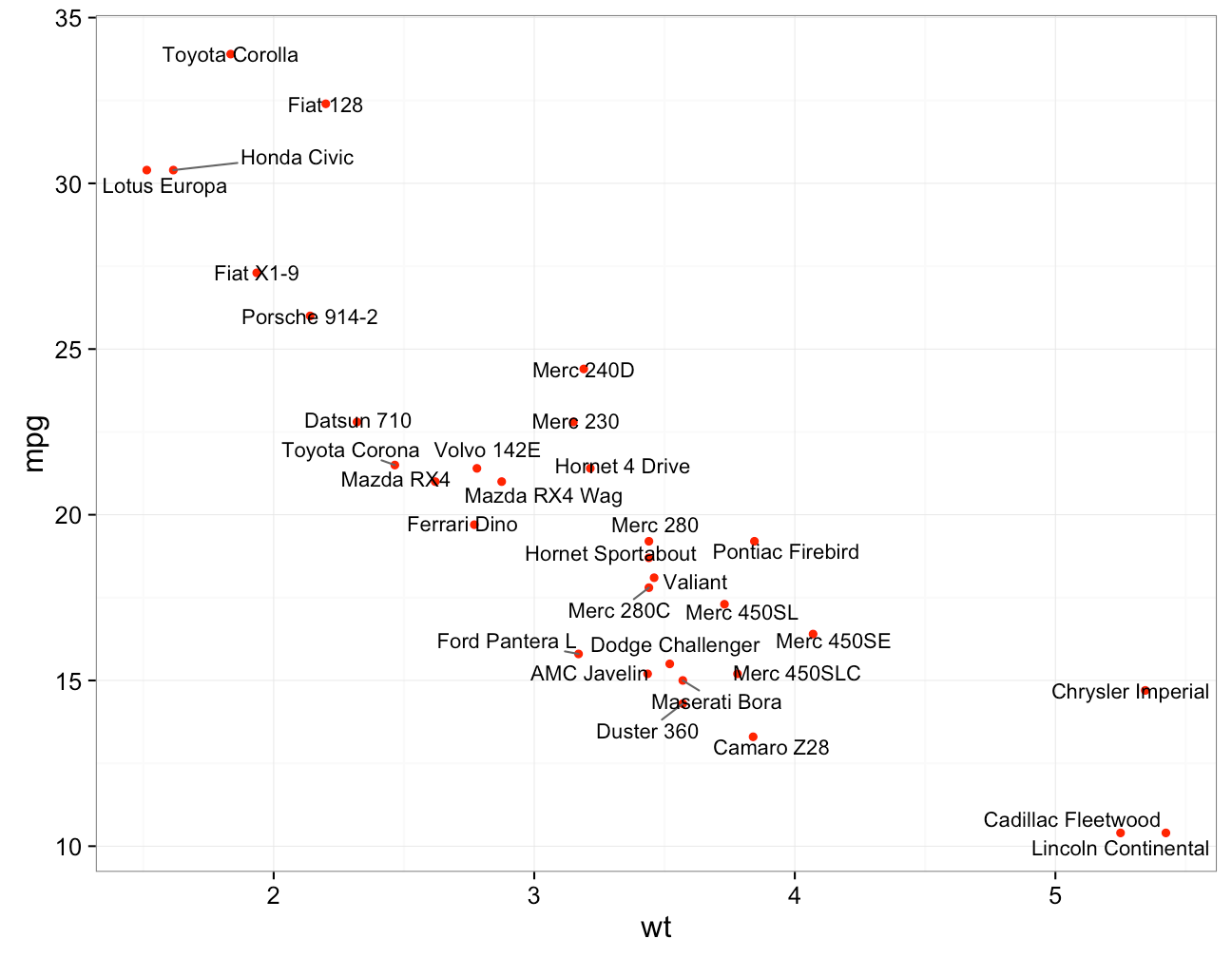### Hide some of the labels

Set some labels to the empty string "" to hide them. All data points will still repel the remaining labels.

set.seed(42)

mtcars$label <- rownames(mtcars) mtcars$label[1:15] <- ""

ggplot(mtcars, aes(wt, mpg)) +
geom_point(aes(color = factor(cyl)), size = 2) +
geom_text_repel(
aes(
color = factor(cyl),
size = hp,
label = label
),
nudge_y = 0.1
) +
theme_bw(base_size = 16)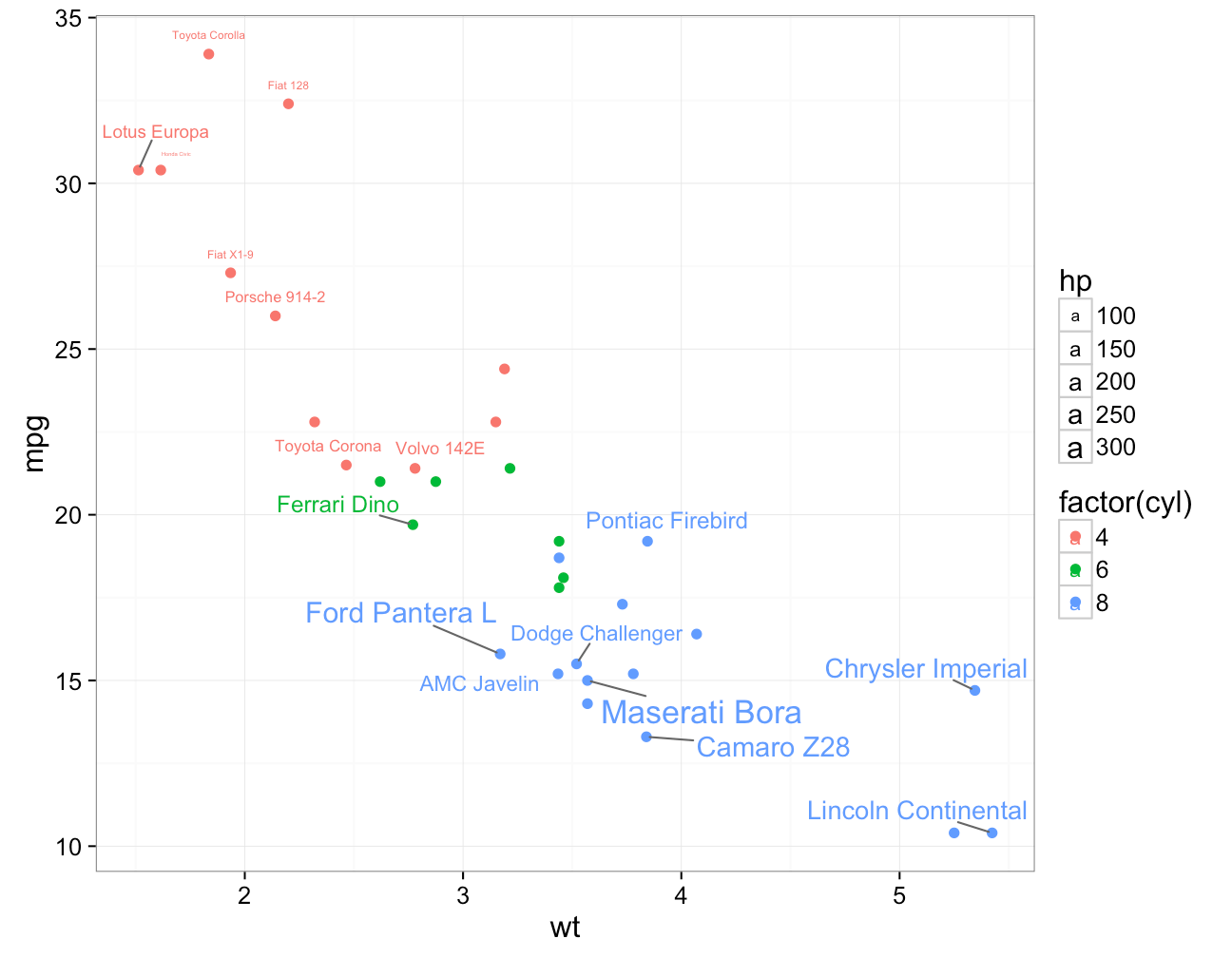### Line plot

set.seed(42)
ggplot(Orange, aes(age, circumference, color = Tree)) +
geom_line() +
coord_cartesian(xlim = c(min(Orange$age), max(Orange$age) + 90)) +
geom_text_repel(
data = subset(Orange, age == max(age)),
aes(label = paste("Tree", Tree)),
size = 6,
nudge_x = 45,
segment.color = NA
) +
theme_classic(base_size = 16) +
theme(legend.position = "none") +
labs(title = "Orange Trees", x = "Age (days)", y = "Circumference (mm)")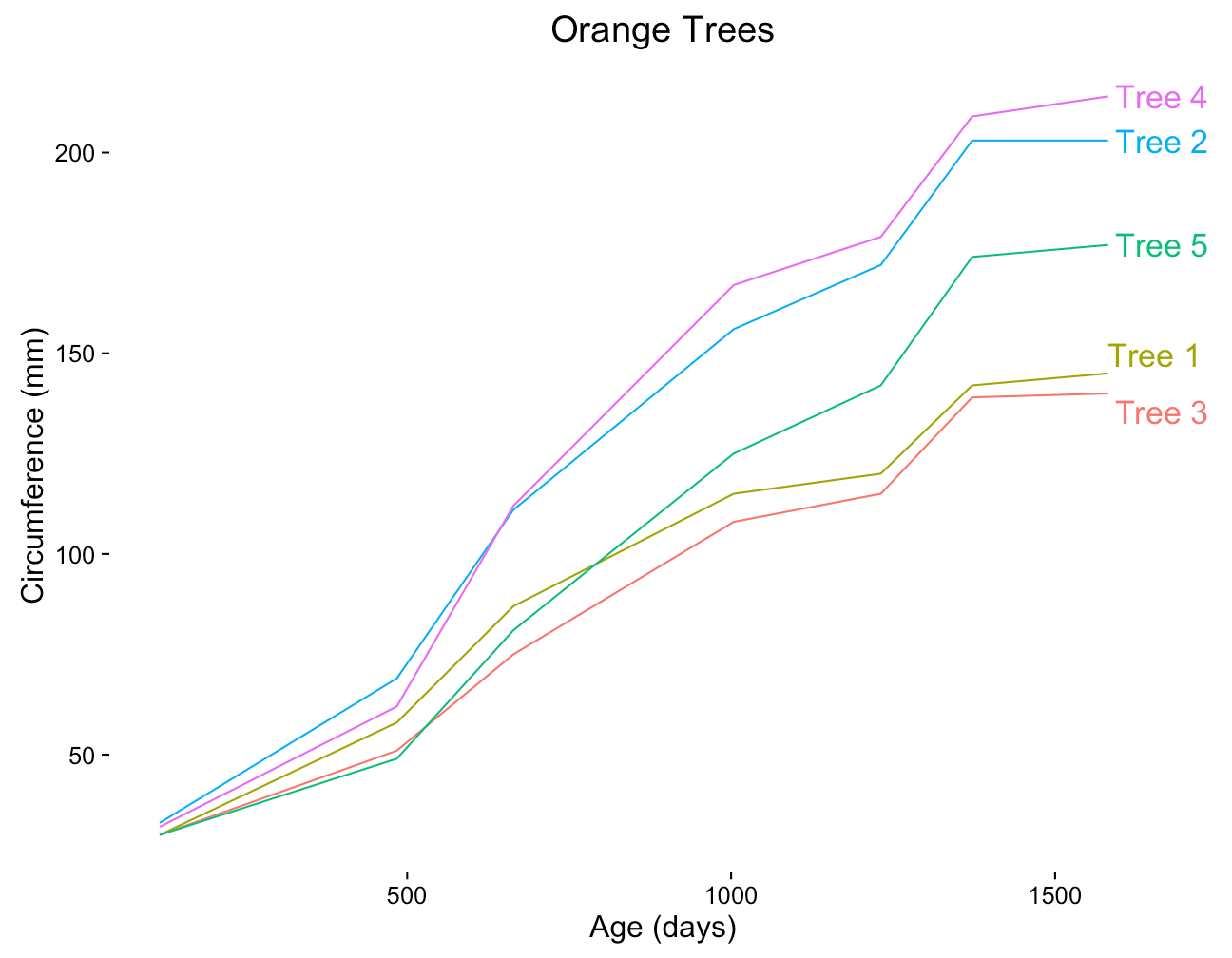### Volcano plot

set.seed(42)

genes$Significant <- ifelse(genes$padj < 0.05, "FDR < 0.05", "Not Sig")

ggplot(genes, aes(x = log2FoldChange, y = -log10(pvalue))) +
geom_point(aes(color = Significant)) +
scale_color_manual(values = c("red", "grey")) +
theme_bw(base_size = 16) +
geom_text_repel(
data = subset(genes, padj < 0.05),
aes(label = Gene),
size = 5,
)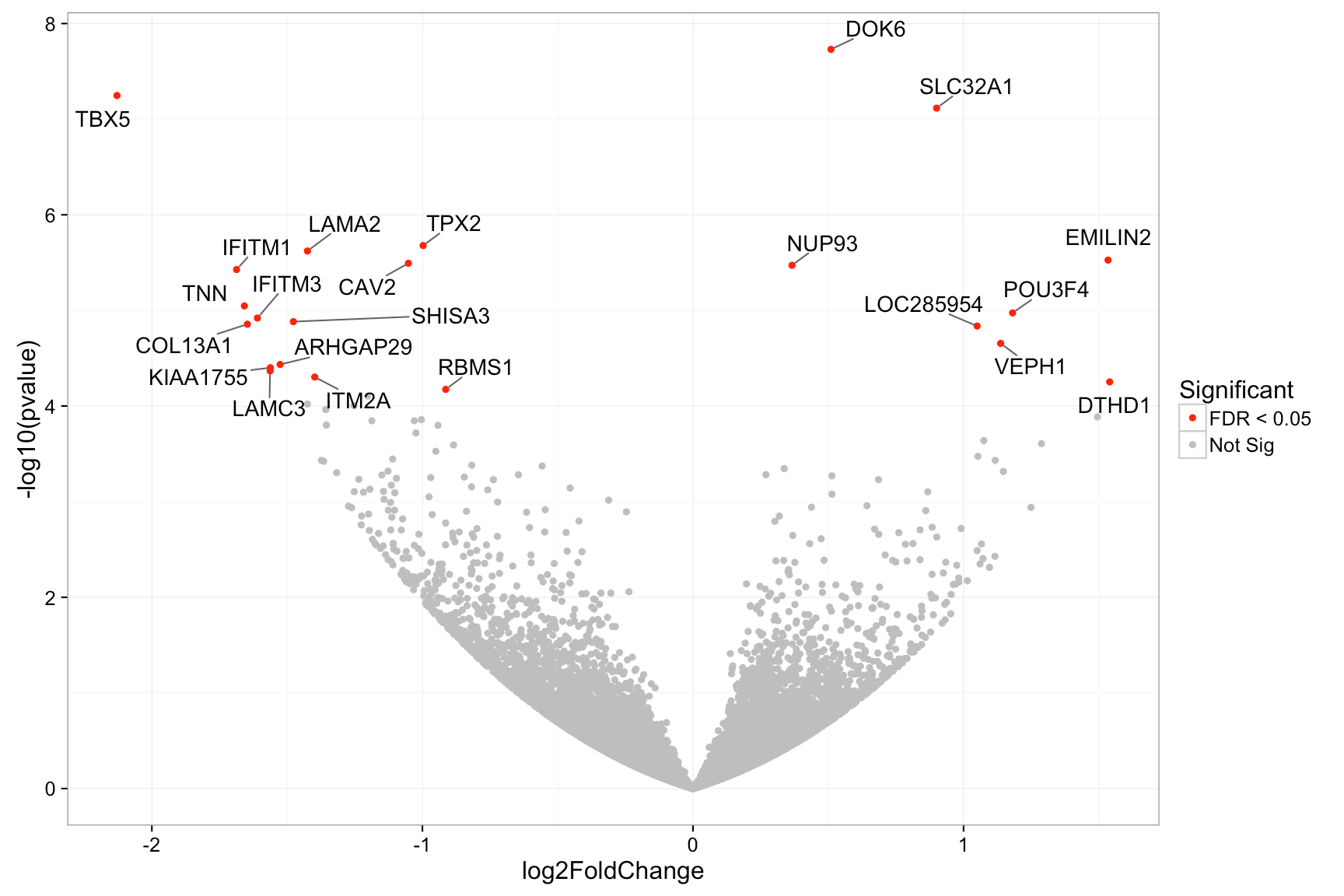### Animation

# This chunk of code will take a minute or two to run.
library(ggrepel)
library(animation)

plot_frame <- function(n) {
set.seed(42)
p <- ggplot(mtcars) +
geom_point(aes(wt, mpg), color = 'red') +
geom_text_repel(
aes(wt, mpg, label = rownames(mtcars)),
size = 5, force = 3, max.iter = n
) +
theme_classic(base_size = 16)
print(p)
}

saveGIF(
lapply(ceiling(1.75^(1:12)), function(i) {
plot_frame(i)
}),
interval = 0.20,
ani.width = 800,
ani.heigth = 600,
movie.name = 'animated.gif'
)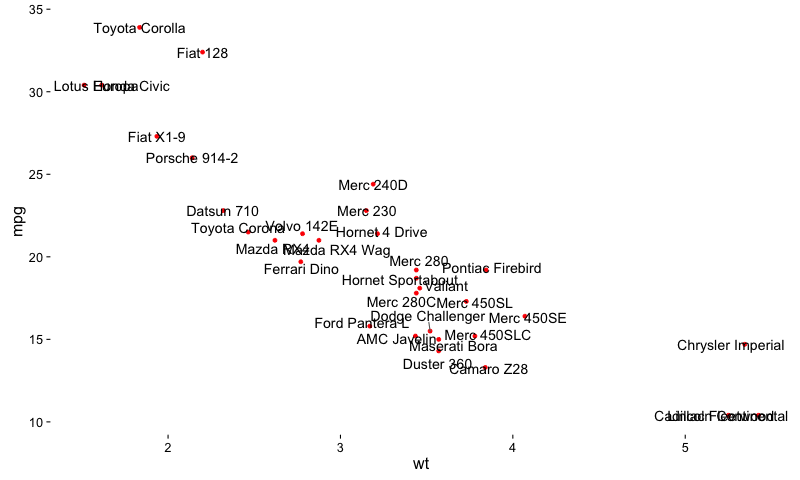## R Session Info

sessionInfo()
## R version 3.2.3 (2015-12-10)
## Platform: x86_64-apple-darwin13.4.0 (64-bit)
## Running under: OS X 10.10.5 (Yosemite)
##
## locale:
##  en_US.UTF-8/en_US.UTF-8/en_US.UTF-8/C/en_US.UTF-8/en_US.UTF-8
##
## attached base packages:
##  stats     graphics  grDevices utils     datasets  methods   base
##
## other attached packages:
##  ggrepel_0.6.3 ggplot2_2.1.0 knitr_1.13
##
## loaded via a namespace (and not attached):
##   Rcpp_0.12.7      codetools_0.2-14 digest_0.6.10    grid_3.2.3
##   plyr_1.8.4       gtable_0.2.0     formatR_1.2.1    magrittr_1.5
##   evaluate_0.8     scales_0.4.0     stringi_1.1.1    labeling_0.3
##  tools_3.2.3      stringr_1.1.0    munsell_0.4.3    colorspace_1.2-6